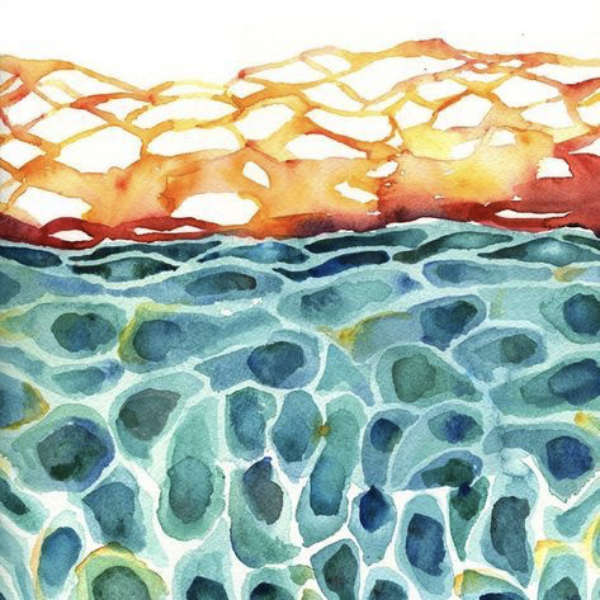# Networks with arbitrary edge multiplicities

Edge multiplicity—the number of triangles attached to edges—is a powerful analytic tool to understand and generalize network properties.

EPL 97, 1 (2012)

V. Zlatić, D. Garlaschelli, G. CaldarelliOne of the main characteristics of real-world networks is their large clustering. Clustering is one aspect of a more general but much less studied structural organization of networks, i.e. edge multiplicity, defined as the number of triangles in which edges, rather than vertices, participate. Here we show that the multiplicity distribution of real networks is in many cases scale free, and in general very broad. Thus, besides the fact that in real networks the number of edges attached to vertices often has a scale-free distribution, we find that the number of triangles attached to edges can have a scale-free distribution as well. We show that current models, even when they generate clustered networks, systematically fail to reproduce the observed multiplicity distributions. We therefore propose a generalized model that can reproduce networks with arbitrary distributions of vertex degrees and edge multiplicities, and study many of its properties analytically.

## More in Structure of how things relate

• Nature Reviews Physics

### Physics of networks

Statistical physics harnesses links between maximum entropy and information theory to capture null model and real-world network features.

• Journal of Statistical Physics

### From ecology to finance

Bipartite networks model the structures of ecological and economic real-world systems, enabling hypothesis testing and crisis forecasting.

• Social Informatics

### Scales in weighted networks

Information theory fixes weighted networks’ degeneracy issues with a generalisation of binary graphs and an optimal scale of link intensities.

• Physical Review E

### Weighted network evolution

A statistical procedure identifies dominant edges within weighted networks to determine whether a network has reached its steady state.